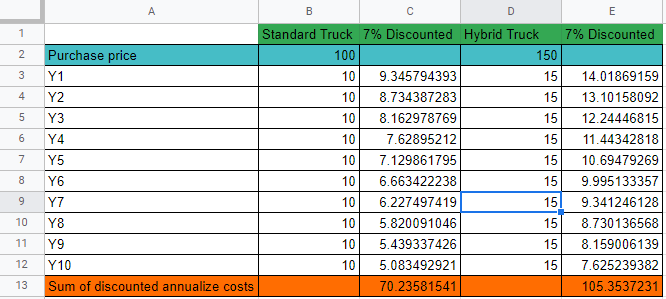# Does total investment include also purchase price and depreciation?

Net Present Value Valuation
New answer on Sep 15, 2020
727 Views

Hello,

A logistics company has to choose between "standard trucks"(ST) and "hybrid trucks"(HT) and has the following data:
----------------------------------ST------------------------HT
1. Purchase price: ____\$100k___________\$150k
2. Maintenance/Yr: _____\$5k_____________\$8k
3. Insurance/Yr:________\$2k_____________\$3k
4. Fuel/Yr:______________\$18k____________\$9k
5. Lifespan: ____________10 Yrs__________10 Yrs
6. Depreciation/Yr: _____\$10k____________\$15k

So far:
operating costs = \$35k/Yr for both ST & HT (maintenance + insurance + fuel + depreciation)
op costs for 10 yrs = \$350k for the 10Y lifespan

In this case, depreciation is the annualized cost (used value of the asset) which after the 10Y period will be less than the initial price.

100k in Year1 > 10k from Y1 to Y10, so depreciation cannot include financing/loan premiums.

How do we include the full purchase price (initial investment or financing fees) in the total investment required while still having the depreciation in mind?

LE:So basically, the depreciation accounts for way less than the purchase price (assuming a 7% discount rate for future cash flows)

(edited)

• Date ascending
• Date descending

Hi there!

Agree with other comments and would approach it also from a cash flow point of view.

In any case, the math of a typical MBB case is not this long and more straightforward.

Cheers,

Clara

Agree with you that the cash flow is the best approach. What about the table? Depreciation is less than the purchase price.... How can you solve that?

Hi,

You will have an initial upfront investment of \$100K and then annual depreciation of \$10K as well as other annual costs. Based on that you calculate the cash flows, discount them and compare between the two options

Best

The depreciation is the annualized cost for the upfront investment, so if you add the yearly costs including depreciation, you have the upfront costs included already. Adding them would be double-counting.

And because the total depreciation over 10 years is equal to the total investment, you also don't need to consider any salvage value at the end of the investment horizon.

Thank you for your answer Henning, it made things clearer. I'm still a bit confused by the premiums for financing. Are we assuming that the depreciation includes both annualized costs and financing premiums? 100k today isn't equal to 10k/Yr. for 10 Yrs.

(edited)

i would consider this as a cashflow problem.

will the \$50 in extra capex (\$150-100) eventually pay off over the investment horizon?

assuming 33% tax rate:

ST outlays:18+2+5-3,3(tax shield from depreciation): ~22

HT outlays:9+3+8-5=~15

you would have a net gain of ~7 if you go for HT per year. depending on the client's wacc, it would make sense the extra invstment :)

btw: equipment financing is not included in this analysis, but it would be eventually addressed in the wacc/irr calculation. if the irr is higher than the cost of financing (assuming 100% debt financing), it would make sense to finance the most expensive equipment (HT)

Thank you for your response. I'm not sure I can follow, in this case assuming the tax deduction 0 and having just that existing data, how do we include the depreciation? 100k today> 10k/yr for the next 10 yrs, so knowing this does the depreciation stand just for the annualized cost? and how can we include the financing premiums to pay the actual price of the trucks?

Also: in this case we don't have enough info to calculate wacc and irr, so the best way is to calculate the savings in annual operating costs and somehow compare with the depreciation and the purchase price (financing options)

assuming no tax credit for depreciation, you wouldnt include it in your cf analysis (dep is a non-cash charge). Thus, you would have to compare the \$5/year net gain (HT vs ST opex) with the \$50 (HT vs ST capex). Like you said, \$50 today > \$5/year for the next ten years, so you would opt for the ST equipment

i would be wary to assume no tax credit for depreciation, though.... thats the main reason why companies capitalizes assets

Hi Mihai,

Just to add to the other answers, here the clear decision point is around time value of money AND cashflows.

Cashflow is super important for companies (and often overlooked). So, you need to recognize that, while these seem like "equal" decisions, ST is the better one as you do not have \$50k tied up in cash over this time period (both limiting total cashflow AND sitting there earning 0% returns)